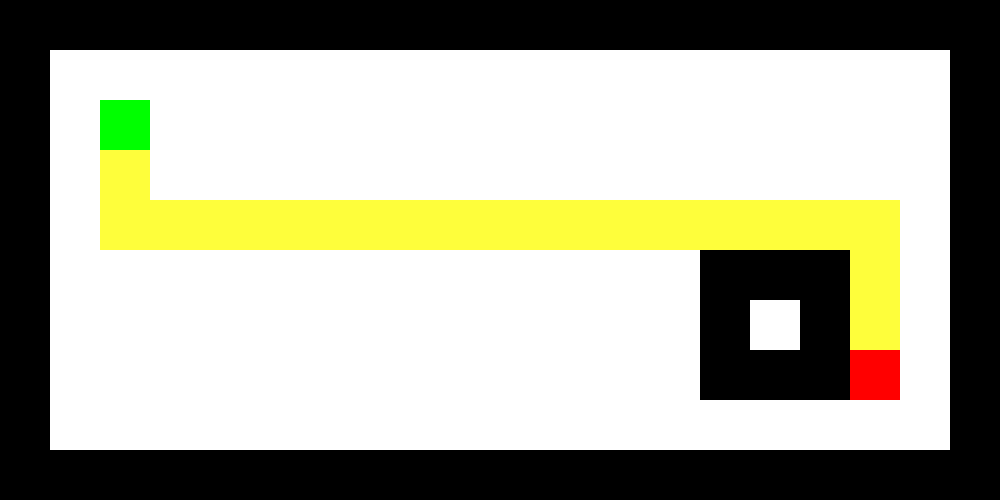# Extra: Viz¶

There are a few utility functions used throughout this jupyter notebook to visualize our grid. We will discuss them briefly here.

In :
```%reload_ext autoreload

import sys
import numpy as np
from pathfinding import grid, utils, finder
from pathfinding.grid import core, viz

# we do this so np print won't truncate
np.set_printoptions(threshold=sys.maxsize)

# let's alias core.find_walkable_neighbors to fwn for brevity
fwn = core.find_walkable_neighbors
```

## Text¶

The first one we see is `generate_text`, which pretty prints our grid.

In :
```polygons = [[(14, 5), (14, 7), (16, 7), (16, 5)]]
maze, start, end = grid.generate(20,10, polygons), (2, 2), (20 - 3, 10 - 3)
path, expansion = finder.bfs(maze, fwn, start, end, with_expansion=True)

print(viz.generate_text(maze, start, end, path))
```
```[[= = = = = = = = = = = = = = = = = = = =]
[=                                     =]
[=   S                                 =]
[=   *                                 =]
[=   * * * * * * * * * * * * * * * *   =]
[=                           = = = *   =]
[=                           =   = *   =]
[=                           = = = E   =]
[=                                     =]
[= = = = = = = = = = = = = = = = = = = =]]
```

For `generate_text`, we only copy the grid/list and replae the values with our own visual text representation (i.e. space for 1)

In :
```def generate_text_map(x):
if x == 0:
return "="
elif x == 1:
return " "
if x == 4:
return "*"
if x == 5:
return "@"
elif x == 2:
return "S"
elif x == 3:
return "E"
else:
return str(x)

def generate_text(grid, start, end, path=[], expansion=[]):
maze = np.copy(grid)

for coord in expansion:
maze[coord][coord] = 5

for coord in path:
maze[coord][coord] = 4

maze[start][start] = 2
maze[end][end] = 3

return np.array2string(maze, formatter={'int': generate_text_map})
```

## Image¶

Image is a bit more involved, but not much harder. Here, we simply create a RGB map from our grid/maze data, and create an image from our rgb array.

In :
```def generate_image(grid, start, end, path=[], expansion=[]):
r = g = b = (grid * 255).astype(np.uint8)
img_array = np.empty((grid.shape, grid.shape, 3), dtype=np.uint8)
img_array[..., 0] = r
img_array[..., 1] = g
img_array[..., 2] = b
for coord in expansion:
img_array[coord, coord] = [200, 200, 200]
for coord in path:
img_array[coord, coord] = [254, 254, 59]
# display start
img_array[start, start] = [0, 255, 0]
# display end
img_array[end, end] = [255, 0, 0]
return img_array

def render_image(img_array, display_size=(1000, 500)):
return display(Image.fromarray(img_array, 'RGB').resize(display_size))
```
In :
```polygons = [[(14, 5), (14, 7), (16, 7), (16, 5)]]
maze, start, end = grid.generate(20,10, polygons), (2, 2), (20 - 3, 10 - 3)
path, expansion = finder.bfs(maze, fwn, start, end, with_expansion=True)

utils.render_image(viz.generate_image(maze, start, end, path))
```## Animation¶

Animation involves the most 'hacks' but not by much. Here, we simply use `matplotlib`'s `FuncAnimation` and loop through drawing `patches.Rectangle`

Note that since most of our algorithms have really large number of nodes expanded, we have to be smart and bundle some nodes together in frames, otherwise it would take a really long time to generate an animation (hence the `frames` argument)

In :
```def generate_anim(grid, start, end, path=[], expansion=[], frames=15):
fig, ax = plt.subplots()

img = generate_image(grid, start, end, path, expansion[:1])
ax.imshow(img)

squares = [Rectangle((coord - 0.5, coord - 0.5), 1, 1, color='black') for coord in expansion]
for sq in squares:
sq.set_alpha(0)

multiple = int(len(expansion) / frames)

def init():
return squares

def update(i):
for j in range(i * multiple, (i + 1) * multiple):
squares[j].set_alpha(0.3)
return squares

return animation.FuncAnimation(fig, update, frames, blit=True, interval=100, init_func=init)
```
In :
```polygons = [[(14, 5), (14, 7), (16, 7), (16, 5)]]
maze, start, end = grid.generate(20,10, polygons), (2, 2), (20 - 3, 10 - 3)
path, expansion = finder.bfs(maze, fwn, start, end, with_expansion=True)

utils.render_anim(viz.generate_anim(maze, start, end, path, expansion, frames=len(expansion)))
```
Out:

Once Loop Reflect

# That's it, thanks for reading!¶

Back to Index

This is a post in the CS 180 Pathfinding series.
Other posts in this series:

### Arian Valdez @Secretmapper

React.JS and Node.JS Software engineering consultant. Developer/Designer Hybrid. Author of Alt Tracker, Combustion, Riyu, etc.